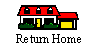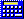RhinoV3 & V4 : Rhino command prompt expression evaluator / calculator and  unit converter (ecncRHCalc)  Calculate common expressions in Rhino command prompt including unit converters, remembers result for the following Analyze commands: Length, Distance, Radius, Angle, Area and Volume and much more ...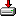Download V4.3 for RhinoV4 ecncRHCalc    ecncRhcalc plug-in commands:  ecncCalcReg ; ecncCalcPrm ecncRhcalc scripting command: CalcExpression (stExpression) Download V2.2 for RhinoV3Download ecncRHCalc (Win2000 and XP)Download ecncRHCalc (Win98 Second Edition)Download ecncRHCalc (Win2000 and XP) newer version

Rhino calculator features: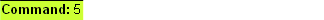*  Supported  operators
 Supported operators: - Logical and and - Logical or or - Less or equal <= greater or equal >= - Not equal != - Equal = = - Greater than > Less than < - Addition and subtraction +, - - Multiplication and division *, / - Exponentiation (raise x to the power of y) ^ It also recognizes the following functions: ABS, EXP, FIX, INT, RND, SNG SIN, COS, TAN, ATAN (deg) ASIN, ACOS, ATAN,  SINR, COSR, TANR, ATANR (radians) ASINR, ACOSR, ATANR,  SINH, COSH, TANH, ASINH, ACOSH, ATANH SINHR, COSHR, TANHR, ASINHR, ACOSRHR, ATANHRLOG2, LOG10, LOG (base 10), LN(base2.718..), EXP, SQR, SIGN ABS, MIN, MAX, SUM, AVG Constants: PI=3.14... and ee=2.718... include parentheses for the above functions i.e.Tangent: TAN(45)
 * Unit converter

Converter: ecncRHCalc plug-in is taking in account current working units and converts it accordingly from to:
Inch(in), MM(mm), Meters(m), Feet(ft), Centimeters(cm), Milli(mi), Nano(n), Micro(mc), square meters(sm), square millimeters (smm)...
i.e. type 20in or 10mm or (27+5.4)ft it will be converted from specified units to current units
or type 20in? or 10mm? or (27+5.4)ft? it will be converted from current units to specified units

example: (2*50)/4 or (2*sin(45))/3 or ((2*sin(45))/3)mm or any combination operators and functions separated with parentheses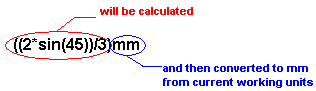* Auto conversions

Type ecncCalcPrm at Rhino command prompt to invoke the following dialog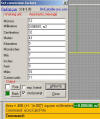ecncRHCalc will monitor Rhino's command prompt and convert Area, Volume, Distance, Radius, Angle and Length values accordingly. i.e. If the current units are millimeters and you want to display area in square meters enter conversion factor under millimeters input box (an area calculated by Rhino will be multiplied with this conversion factor), enter text that you would like to appear at the end of converted number (separated with , or ;) and click on UPDATEFormat for conversion factor: Area factor; msg; Volume factor; msg; Distance factor; msg; Radius factor; msg;  Angle factor; msg;  Length factor; msg;  in short 1; area;2; volume;3; distance;4; radius;5; angle;6; length  i.e. 0.831;m^2;0.5; m^3;25.4; in;4; Radius;5; Angle;6; Length Command history window (F2) is updated as well

 * Last calculated value

TABs invokes last numerical value shown in the command prompt.
- ecncRHCalc remembers result for the following Analyze commands: Length, Distance, Radius, Angle, Area and Volume
- just invoke one of this commands and then press TAB any time you need last measurement result/input
- if user calculate something (pressing CTRL/CR/SPACEBAR) this will be remembered as a last input

 * Coordinate input

- ecncRHCalc recognizes a comma delimited input i.e.
i.2+3,6*2,9/3 ecncRHCalc will automatically place 5,12,3 before it reaches Rhino input
2+4in,sin(45),15cm : if your default units are mm you'll get the following ecncRHCalc output:103.6,0.7071,150
- ecncRHCalc will recognize world coordinates w, relative coordinates r, world relative coordinates wr and angle prefixes <

 * Settings and HotKeys:

- once user press CTRL or Enter/CarriageReturn (if set) the ecncRHCalc will look at Rhino's command prompt and calculate if there is anything to calculate, otherwise, if ecncRHCalc cannot evaluate an  expression, the expression will be highlighted or set to 0

-  TAB: paste last calculated value at the command prompt.

- press ESC to clear command prompt

-  exp.format: shows output in exponential format.

-  cmd prompt : enable/disable holding last results (press TAB key to paste result into Rhino command prompt).

-  Fore/Back : outputs result in desired color combination.

-  Enable/Disable Space key to evaluate an expression

 * VB/VBS scripting support: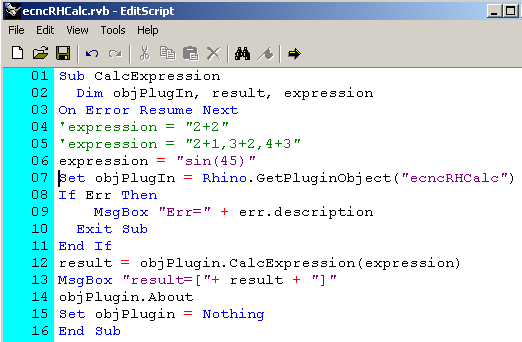* Plug-In Install/Uninstall procedure:

To Install ecncRHCalc:
==================

- Start Rhino
- Install plug-in (Type: PlugInManager in the command prompt then click on INSTALL and browse to ecncRHCalc.rhp
(after this initial install ecncRHCalc will be loaded on Rhino start up)

To Uninstall ecncRHCalc:
===================

- Close Rhinoceros
- Locate ecncRHCalc.rhp on your computer and delete it.Download V4.3 for RhinoV4 ecncRHCalc4    ecncRhcalc plug-in commands:  ecncCalcReg ; ecncCalcPrm ecncRhcalc scripting command: CalcExpression (stExpression) Download V2.2 for RhinoV3Download ecncRHCalc (tested on Win2000 and XP)Download ecncRHCalc (tested on Win98 Second Edition)Download ecncRHCalc (tested on Win2000 and XP)

An older version (unsupported)RhinoV3 SP3 -V4 InLine Calculator - FREE Calculate common expressions in the Rhino's command prompt
 supported operators: - Addition and subtraction +, - - Multiplication and division *, / - Exponentiation ^ - Integer division \ - Mod % It also recognizes the following functions: ABS, EXP, FIX, INT, LOG (base 10), LN(base2.718..), RND, SNG, SQR SIN, COS, TG, ATG (degrees) SINR, COSR, TGR, ATGR(radians) include parentheses for the above functions i.e.Tangent: TG(45)

- Start Rhino
- Start rhinocalc.exe (or assign it to a button NoEcho _Run "path to rhinocalc.exe")
- right mouse click on RhinoCalc icon in the systray for pop-up menu to close calculator, enable it, disable it, test it ...
- it works with one rhino session only (first or last started rhino session)...
- once user press Enter/CarriageReturn, SPACE BAR or CTRL key the RhinoCalc will look at the Rhino's command prompt and calculate if there is anything to calculate!
- indicator that RhinoCalc is running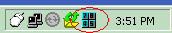i.e. 2+4,6*2,9/3 RhinoCalc will automatically place 6,12,3 before it reaches Rhino input
more examples: (2*50)/4 or (2*sin(45))/3 or any combination operators and functions separated with parenthesesDownload RhinoCalc for RhinoV3 (tested on win2000 and XP)